Core Java - Interview Questions and Answers for 'Factorial' | Search Java Interview Question - javasearch.buggybread.com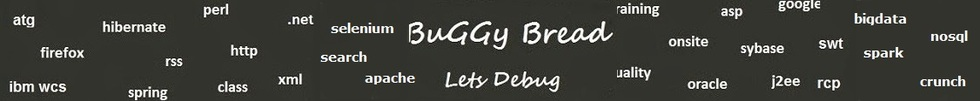# Search Interview QuestionsMore than 3000 questions in repository.There are more than 900 unanswered questions.Have a video suggestion.
Click Correct / Improve and please let us know.
Label / Company      Label / Company / TextSubmit Question

Core Java - Interview Questions and Answers for 'Factorial' - 2 question(s) found - Order By NewestQ1. Write a Program that gets a set of numbers , filters out the non prime numbers , calculate the factorial of each prime number and then finds the average of all factorials using Lambda expressions2017-05-14 14:02:57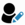public static void main(String args[]) {

// Declare and Initialize the Collection
Set<Integer> intSet = new HashSet<Integer>();

double averageOfNonPrimeFactorials = intSet.stream().filter(p->checkIfPrime(p)).collect(Collectors.averagingInt(p->calculateFactorial(p)));

System.out.println(averageOfNonPrimeFactorials );
}

static private boolean checkIfPrime(int num){
for(int count=2;count < num;count++){
if(num % count == 0){
return false;
}
}
return true;
}

static private int calculateFactorial(int num){
int factorial = 1;
for(int count=num;count > 0;count--){
factorial = factorial * count;
}
return factorial;
}
}Help us improve. Please let us know the company, where you were asked this question :LikeDiscussCorrect / Improvejava 8  lambda expressions  lambda  filter  Collectors  factorial  prime numberRelated Questions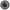What is a Lambda Expression ? What's its use ?Difference between final and effectively final ? Why is effectively final even required ?Difference between Predicate, Supplier and Consumer ?What are the problems one could face while working with serverless technologies like AWS Lambda ?How does AWS Lambda handle failure during event processing?What would you do if you see an error thrown by a lambda function as "Access Denied" while doing something with S3 ?What are the advantages and disadvantages of using Lambda over EC2 ?What are the different trigger types in AWS Lambda ?What does the following lambda expression means ?Difference between DoubleSummaryStatistics , IntSummaryStatistics and LongSummaryStatistics ?Q2. Write a program to calculate factorial of a number using recursionCore JavaAns.

public class Factorial {
public static void main(String[] args){
int x = 5;

System.out.println(calculateFactorial(x));

}

private static int calculateFactorial(int x){
if(x==1){
return 1;
}
return x * calculateFactorial(x-1);
}
}Help us improve. Please let us know the company, where you were asked this question :LikeDiscussCorrect / Improvefactorial  calculate factorial  code  coding  recursion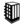Asked in 1 Companies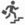basicRelated QuestionsWhat are the steps to be performed while coding Junit with Mocking framework ?What is a Sequence File?What different level of logging you use while coding ?Have you ever encoded the response before sending it back from the service? If Yes , Which encoding was used ?What are the different type of encoding you have used ?How does encoding affect using Reader / writer classes or Stream classes in Java ?What is the difference between html encoding and url encoding ?Does spaces get's encoded in html encoding ?

## Help us and Others Improve. Please let us know the questions asked in any of your previous interview.

Any input from you will be highly appreciated and It will unlock the application for 10 more requests.